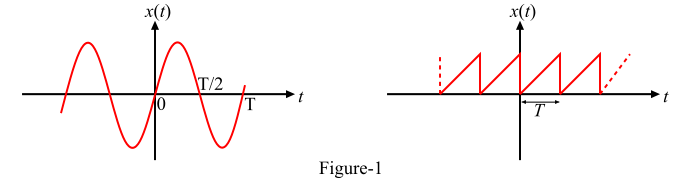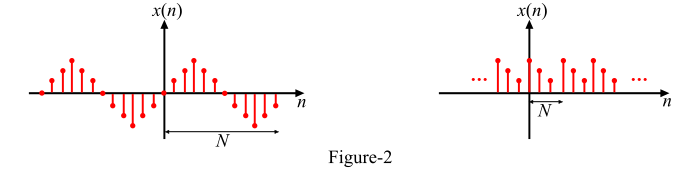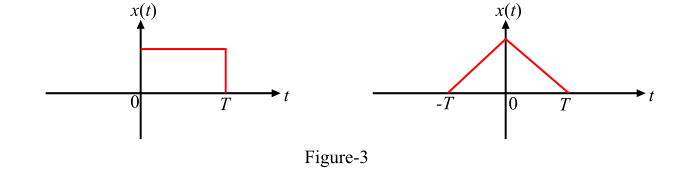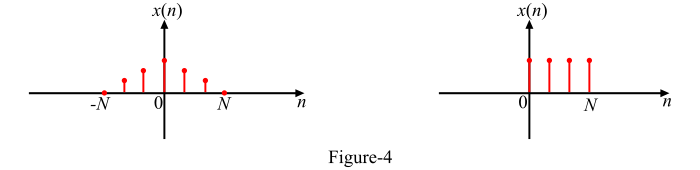# Signals and Systems: Periodic and Aperiodic Signals

A signal is said to be periodic signal if it has a definite pattern and repeats itself at a regular interval of time. Whereas, the signal which does not at the regular interval of time is known as an aperiodic signal or non-periodic signal.

## Continuous Time Periodic Signal

A continuous time signal x(t) is said to be periodic if and only if

𝑥(𝑡 + 𝑇) = 𝑥(𝑡) for − ∞ < 𝑡 < ∞

Where, T is a positive constant that represents the time period of the periodic signal. The smallest value of the time period (T) which justifies the definition of the periodic signal is known as fundamental time period of the signal and is denoted by (𝑇0).

Also,

𝑥(𝑡 + 𝑚𝑇) = 𝑥(𝑡)

Where, m is an integer. This means if the definition is satisfied for 𝑇 = 𝑇0, then it is also satisfied for 𝑇 = 2𝑇0, 𝑇 = 3𝑇0 … and so on with 𝑇0 as the fundamental time period. Therefore, the fundamental time period defines the duration of one complete cycle of the periodic signal x(t).

The reciprocal of the time period (T) of the periodic signal is called the frequency (f) of the signal, i.e.,

$$\mathrm{f=\frac{1}{T}}$$

Since the angular frequency is given by,

𝜔 = 2𝜋𝑓

$$\mathrm{\therefore Time\, period,\: T=\frac{2\Pi }{f}}$$

Some examples of continuous-time periodic signals are shown in Figure-1.## Discrete Time Periodic Signal

A discrete-time signal x(n) is said to be periodic if it satisfies the following condition

𝑥(𝑛) = 𝑥(𝑛 + 𝑁);   for all integers 𝑛

Here, N is the time period of the periodic signal and a positive integer. The smallest value of the time period (N) which satisfies the above condition is known as fundament time period of the signal. The fundamental time period (N) may be defined as the minimum number of samples taken by signal to repeat itself.

The angular frequency of the discrete time periodic sequences is given by,

$$\mathrm{\omega =\frac{2\Pi }{N}}$$

Therefore, the time period of the sequence is,

$$\mathrm{N =\frac{2\Pi }{\omega }}$$

Some examples of the discrete time periodic sequences are shown in Figure-2.## Continuous Time Aperiodic Signal

If a continuous time signal does not have a definite pattern and does not repeat at regular intervals of time is known as continuous time aperiodic signal or nonperiodic signal.

In other words, a signal x(t) for which no value of time t satisfies the condition of periodicity, is known as aperiodic or non-periodic signal.

Some examples of continuous-time aperiodic signals is shown in Figure-3.## Discrete Time Aperiodic Signal

If the condition of periodicity is not satisfied even for one value of n for a discrete time signal x(n), then the discrete time signal is aperiodic or nonperiodic. Examples of discrete time aperiodic signals are shown in Figure-4.## Numerical Example

Examine whether the following signals are periodic or not? If periodic then determine the fundamental period of the signal.

• 𝑥(𝑡) = sin 10𝜋𝑡

• 𝑥(𝑡) = sin 𝜋𝑡 𝑢(𝑡)

### Solution

• Given signal is,

𝑥(𝑡) = sin 10𝜋𝑡

Since the signal x(t) is a sinusoidal signal, hence, it is a periodic signal.

Now, comparing x(t) with standard signal, i.e., sin 𝜔𝑡, we get,

𝜔 = 10𝜋

$$\mathrm{\therefore \: Fundamental \: Period ,T =\frac{2\Pi }{\omega }=\frac{2\Pi }{10\Pi }=\frac{1}{5}\: sec}$$
• Given signal is,

𝑥(𝑡) = sin 𝜋𝑡 𝑢(𝑡)

Here, x(t) is the product of sinusoidal signal (sin 𝜋𝑡) and unit step signal (𝑢(𝑡)). As we know, the signal sin 𝜋𝑡 is periodic with time period 𝑇 = 2𝜋⁄𝜔 while the signal 𝑢(𝑡) exists only for 0 < 𝑡 < ∞. Thus, 𝑢(𝑡) is not a periodic signal.

Therefore, the signal x(t) is an aperiodic or non-periodic signal.